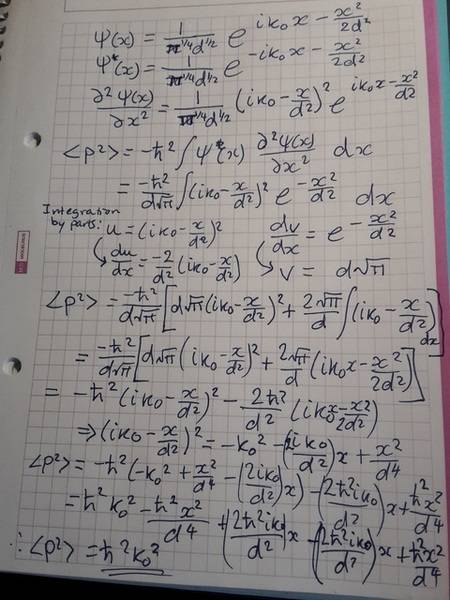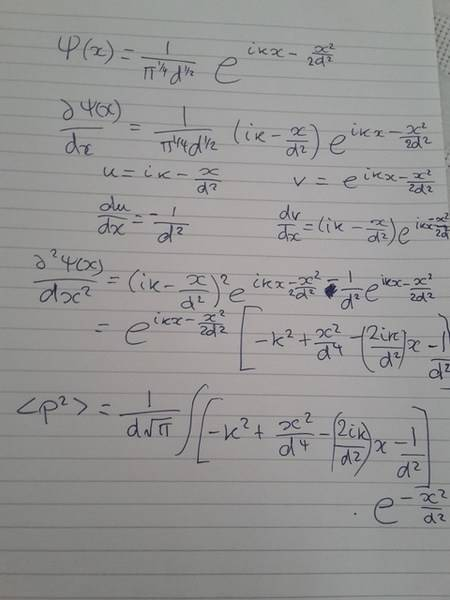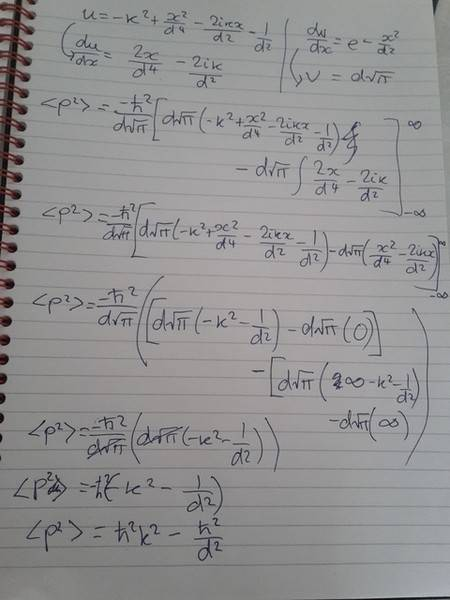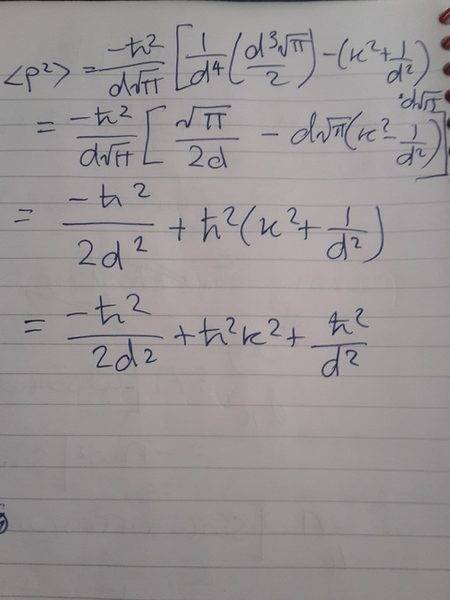# Calculating the momentum operator in a quantum state

DarkMatter5

## Homework Statement

A gaussian wave packet is given by the formula:
Ψ(x)=(1/(π1/4d1/2))eikx-(x2/2d2)

Calculate the expectation value in this quantum state of the momentum squared.

## Homework Equations

<p2>=-ħ∫Ψ*(X) (d2Ψ(x)/dx2) dx
∫e(-x2/d2) dx= d√π
∫xe(-x2/d2) dx =0
∫x2e(-x2/d2) dx = (d3√π)/2

## The Attempt at a Solution

Here is my attempt at a solution.I got ħ2k2. The correct answer is ħ2k2 + ħ2/(2d).
All help is very much appreciated.

Homework Helper
Working in momentum space by first Fourier transforming the given wavefunction might help minimize the possibility of error during calculation.

DarkMatter5
Thank you. Unfortunately I have no idea how to do that. Did you see an error in my calculation?

Homework Helper
I didn't go through the detail but it is strange that you still have ##x## after finishing the integral, although the terms containing it eventually cancel in the final step. You were doing a definite integral over ##x##, so logically this variable should not appear after the integral is evaluated.

DarkMatter5
I was doing a definite integral? Where? I shouldn't be doing definite integrals.

Homework Helper
I was doing a definite integral? Where? I shouldn't be doing definite integrals.
You are, in calculating the expectation value you integrate from ##-\infty## to ##+\infty##.

DarkMatter5
Oh. I thought that because the limits were infinite that it would be an indefinite integral.

Homework Helper
The second derivative of ##\psi(x)## is not correct.

•DarkMatter5
DarkMatter5
Thank you for that correction. e should be to the power of ikx-(x2/2d2.) But it still doesn't change the answer. The mark scheme said that for some reason (ik-(x/d2))2 simplifies to -1/d2. I don't know how this is possible!

Homework Helper
Thank you for that correction. e should be to the power of ikx-(x2/2d2.) But it still doesn't change the answer. The mark scheme said that for some reason (ik-(x/d2))2 simplifies to -1/d2. I don't know how this is possible!
You seem to be not getting the correct second derivative yet. Ok, could you please show how you got the first derivative ##\frac{d}{dx}\psi(x)##?

Homework Helper
Gold Member
2021 Award
Thank you for that correction. e should be to the power of ikx-(x2/2d2.) But it still doesn't change the answer. The mark scheme said that for some reason (ik-(x/d2))2 simplifies to -1/d2. I don't know how this is possible!

You're also missing a trick in that ##\int_{-\infty}^{\infty} x e^{-\lambda x^2} dx = 0##.

You should expand your quadratic term and the term in ##x## will vanish under the integral. That's the simplification you're missing.

But, as @blue_leaf77 says, you need to get your differentiation right first.

DarkMatter5
I tried the derivative again but it doesn't simplify to what you are saying for some reason:Homework Helper
Gold Member
2021 Award
The second derivative looks right. But, you're still taking an indefinite integral for some reason:

##<p^2> =\int_{-\infty}^{\infty} \dots dx##

Which is a number, not a function of ##x##.

•DarkMatter5
DarkMatter5
The final answer should be ħ2k2+(ħ2/(2d2)). But I get the following:Homework Helper
You made a mistake when calculating ##v##. If you want to do this problem via integration by part, then you should have left ##v## as a function, not a number. In other words you shouldn't evaluate it yet with the given limits. I strongly suggest that you compute ##\langle p^2 \rangle## directly term by term using the relations you already have under the relevant equations part.

DarkMatter5
They gave me the equation ∫e(-x2/d2) dx= d√π. That is what I used to calculate v. I'm not sure how to get the other equations in my working out. I don't get ∫xe(-x2/d2) dx =0 anywhere in my answers so I can' use it.

Homework Helper
∫e(-x2/d2) dx= d√π.
That's resulting in a number, right? Not a function, as how ##v(x)## should be.
You have to solve the following integral
$$\langle p^2 \rangle = \frac{-\hbar^2}{d\sqrt{\pi}} \left( \frac{1}{d^4}\int_{-\infty}^\infty x^2 e^{-x^2/d^2} dx - \left(k^2+\frac{1}{d^2}\right) \int_{-\infty}^\infty e^{-x^2/d^2} dx - \frac{2ik}{d^2} \int_{-\infty}^\infty x e^{-x^2/d^2} dx \right)$$
You can completely compute those three integrals using the formula in your original post.

•DarkMatter5 and PeroK
DarkMatter5
Hmm okay. How did you derive that integral? I'm confused as to how you got that equation.

Homework Helper
Hmm okay. How did you derive that integral? I'm confused as to how you got that equation.
You derived the integral, at the bottom of the image in post #12. I merely rearrange the terms and add ##-\hbar^2## and the necessary integration element ##dx##.

•PeroK
DarkMatter5
Sorry, I really am not understanding this. Please could you explain how my equation turns into the integral? I can't figure out how to get from what I wrote in post #12 to the integral. I don't think I was taught how to do that.

Homework Helper
I am personally really surprised that you cannot recognize two identical equations with only the terms being rearranged while the other part of you are able to do the more complicated and lengthy differentiations involving product of functions when you correctly calculate ##\frac{d^2}{dx^2} \psi(x)##. It's just rearranging the terms and taking out constants (i.e. anything not containing ##x##) outside the integral, and also making use of the fact that integral is a linear operation ##\int (f(x) + g(x))dx = \int f(x) dx + \int g(x) dx##.

DarkMatter5
Oh so you multiplied the bracket by the exponent function and then the linear operation of integrals. I see that makes sense. Thank you.

And yes, I am not a natural at mathematics. I only got this far by determination and I still find simple mathematical concepts challenging sometimes and need slow patient explanations.

Almost there. I got this answer:•